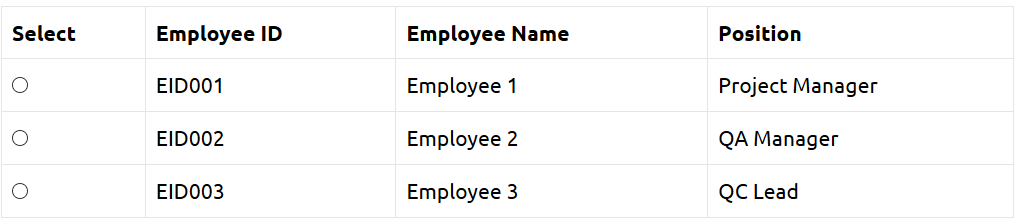# How To Handle Web Tables in Selenium Python

In the previous articles on Selenium Python Tutorial, we have covered “Exceptions in Selenium Python“. In this tutorial, we will learn How To Handle Web Tables in Selenium Python.

A web table in an HTML document is defined under <table> tag. The rows of a table are represented with the <tr> tag and the columns in a table are represented with the <td> tag. Mostly each table has headers represented with the <th> tag.

Let us consider the below table for our discussion.To work with a web table, we should be able to handle scenarios like how to fetch the row numbers, column numbers, a particular cell value, fetch all cell values in a row, fetch all cell values in a column, fetch all the cell values and so on.

To calculate the total number of rows, we shall first create a customized xpath to represent all rows in a table with the help of find_elements_by_xpath () method. Since this method returns a list, we can obtain the row count with the help of len method.

Code Implementation to calculate row count in a table.

To calculate the total number of columns, we shall first create a customized XPath to represent all rows in a table with the help of find_elements_by_xpath () method. Since this method returns a list, we can obtain the row count with the help of len method. We have to fix a row number before calculating the column count with <td>. If we set row 1, we have to use <th> for the columns. For any other row except 1, we have to use <td>.

Code Implementation to calculate column count in a table.

To get the headers in a table, we shall first create a customized XPath to represent all rows in a table with the help of find_elements_by_xpath () method. Since this method returns a list, we can obtain the row count with the help of len method. We have to iterate through row number 1, then move to <th>. Once we get hold of the first row, we shall use the text method to get all the table headers.

Code Implementation to get the table headers.

To get all the cell values of a particular row, we shall first create a customized XPath to represent all cell data of a row in a table with the help of find_elements_by_xpath () method. Since this method returns a list, we can obtain the row count with the help of len method. We have to iterate through the columns of a particular row number [greater than 1], having <td> tag. Once we get hold of all the values in that particular row, we shall use the text method to get all the cell data.

Code Implementation to get cell data of a particular row.

To get all the cell values of a particular column, we shall first create a customized XPath to represent all cell data of a column in a table with the help of find_elements_by_xpath () method. Since this method returns a list, we can obtain the row count with the help of len method. We have to iterate through the rows of a particular column number, having a <tr> tag. Once we get hold of all the values in that particular column, we shall use the text method to get all the cell data.

Code Implementation to get cell data of a particular column.

To get all the cell values of a table, we shall first create a customized XPath to represent all the rows and columns in a table with the help of find_elements_by_xpath () method. Since this method returns a list, we can obtain the row and column count with the help of len method. We have to iterate through each row and each column of a particular table then fetch the cell data with the text method.

Code Implementation to get all the cell data in a table.

To verify if a particular text exists in the table, we shall first create a customized xpath to represent all the rows and columns in a table with the help of find_elements_by_xpath () method. Since this method returns a list, we can obtain the row and column count with the help of len method.

We have to iterate through each row and each column of a particular table then fetch the cell data with the text method. Once the cell data is fetched, we shall verify if it matches with the text we are looking for with the help of text () function in xpath.

Code Implementation to search a specific text in a table.

To get value from a specific cell in a table, we shall first create a customized XPath to identify the cell with the help of find_element_by_xpath () method

Code Implementation to get the value from a particular cell.

In the next article, we will learn Handling cookies in Selenium Python

Related posts:

Get our latest blog posts delivered to your inbox

Subscribe and get popular blog posts about software testing industry.YOU MAY ALSO ENJOY THESE ARTICLES